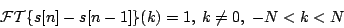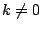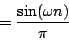Next: Parabolic wave Up: Fourier series of the Previous: Fourier series of the   Contents   Index

## Sawtooth wave

First we apply this to the sawtooth wave. Forwe have:Ignoring the constant offset of, this gives an impulse, zero everywhere except one sample per cycle. The summation in the Fourier transform only has one term, and we get:We then apply the difference formula backward to get:valid for integer values of, small compared to, but with. (To get the second form of the expression we plugged inand.)

This analysis doesn't give us the DC component, because we would have had to divide by. Instead, we can evaluate the DC term directly as the sum of all the points of the waveform: it's approximately zero by symmetry.

To get a Fourier series in terms of familiar real-valued sine and cosine functions, we combine corresponding terms for negative and positive values of. The first harmonic () is:and similarly theth harmonic isso the entire Fourier series is:Next: Parabolic wave Up: Fourier series of the Previous: Fourier series of the   Contents   Index
Miller Puckette 2006-12-30